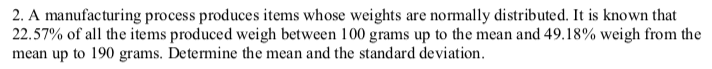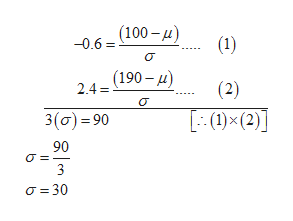# 2. A manufacturing process produces items whose weights are normally distributed. It is known that22.57% of all the items produced weigh between 100 grams up to the mean and 49.18% weigh from themean up to 190 grams. Determine the mean and the standard deviation.

Question
23 viewshelp_outlineImage Transcriptionclose2. A manufacturing process produces items whose weights are normally distributed. It is known that 22.57% of all the items produced weigh between 100 grams up to the mean and 49.18% weigh from the mean up to 190 grams. Determine the mean and the standard deviation. fullscreen
check_circle

Step 1

2.

You use z=(x-mu)/sigma twice and get two linear equations which you can then solve for µ and σ.
One equation: 0.2257 units of area between z and 0, so 0.5000 – 0.2257 = 0.2743 area to the left of z. Use a table of values or a stats calculator to find z= –0.6 approximately. Substitute z= –0.6 and x=100 into z=(x-mu)/sigma.

Second equation: area 0.4918 from mean (z=0) to 190. Area to left is 0.5+0.4918 = 0.9918 which gives a z value of z=2.4 approximately. Substitute z=2.4, x=190 into z=(x-mu)/sigma.

Two equations in two unknowns , use linear algebra to solve:help_outlineImage Transcriptionclose(100– 4) (1) -0.6 = (190 – u) (2) 2.4 = [:(1)×(2)] 3(0) = 90 90 3 O = 30 fullscreen
Step 2

The value of m...

### Want to see the full answer?

See Solution

#### Want to see this answer and more?

Solutions are written by subject experts who are available 24/7. Questions are typically answered within 1 hour.*

See Solution
*Response times may vary by subject and question.
Tagged in

### Other